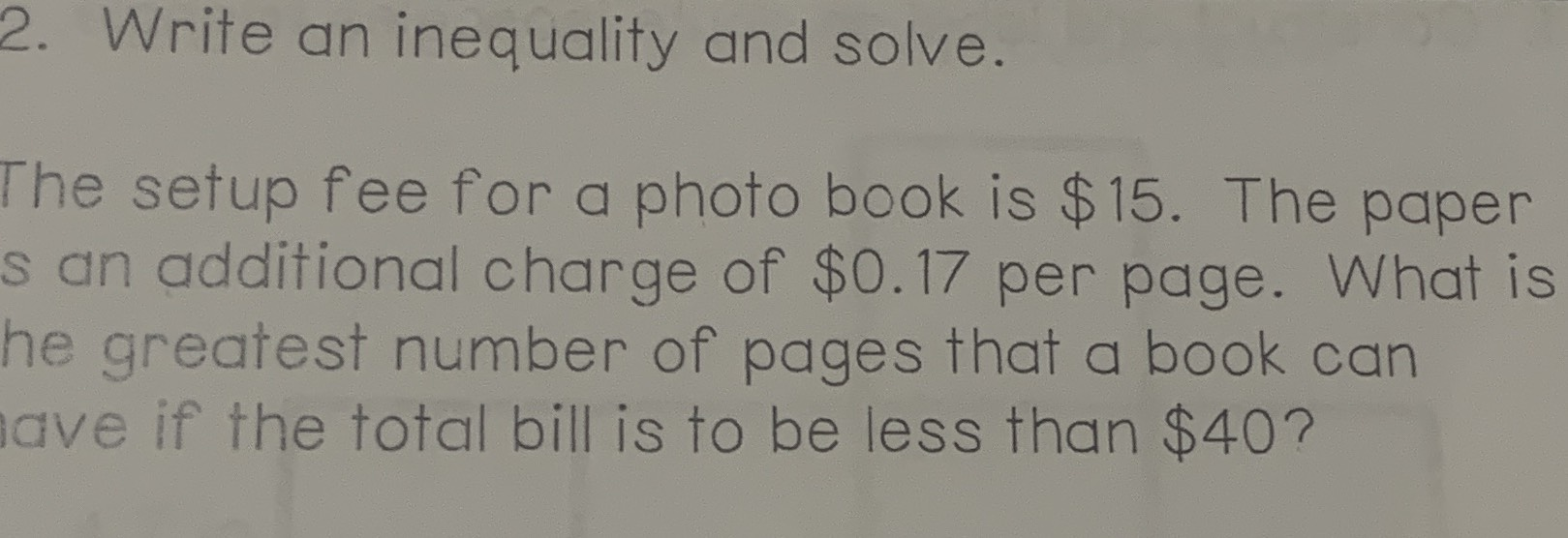### ¿Todavía tienes preguntas de matemáticas?

Pregunte a nuestros tutores expertos
Algebra
Pregunta2. Write an inequality and solve. The setup fee for a photo book is $$\ 15$$ . The paper s an additional charge of $$\ 0.17$$ per page. What is he greatest number of pages that a book can ave if the total bill is to be less than $$\ 40$$ ?

$$15+ 0.17x< 40$$